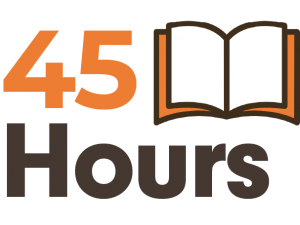# Vedic Maths Course for Competitive Exams

## Top 6 ways on how Vedic Maths can help you crack that Exam

### Focus on the Concept of Simplicity

Use of simple principles to get solutions for complex problems

### Improve Precision by Mental Calculation

Vedic Math makes you sharper and faster in the long run

### Improve stamina & reasoning skills

Builds your Stamina for Quantitative Aptitude and Reasoning

An alternative calculating method to crack your coveted exam

### Beat your Competition with ease

Stand out from the rest. Make Vedic Math your winning Edge!

### Winner’s Choice

Winner's always go the extra mile and that’s why they study Vedic Math.

## Race against Time

Every competitive exam out there is essentially a race against time. There are a ton of questions and one is expected to maximize the number of questions answered with cent per cent accuracy or face negative marking. A competitive exam is like being on a battlefield and trying to use every weapon and strategy you got to win. Vedic Math is like that. It is a weapon you can use to win your war against that competitive exam in a stressed-out environment, where every second matters.

Traditional ways take its own time to be applied and you need out of box
solutions and quick thinking to crack different types of Math problems. A lot can be against you if you are not well prepared to win. From huge competition to the lack of Math skill to even exam related stress and anxiety.

## How do you improve your odds

You certainly need able mentors, guides and strategies to help you out. The Vedic Maths Forum India can help you crack the Math section of your examination be it the Common Admission Test (CAT) or the GMAT to get into a Business School or the SAT to get into a premier ivy league university of your choice. It’s one of a kind course where you learn new methods and concepts to crack that coveted examination.## 45 Hours of Fundamentals in Vedic Maths

The Course has been neatly divided into various relevant topics and contains clear introduction on different mathematical topics, the important derivations and formulae that aid in understanding the core concept of the subject and solve the questions faster yet correctly. Vedic Math helps in quant concepts such as number systems, data interpretation and even reasoning to some extent.

## What do you get?

This course is rigorous for 45 hours and guarantees a better understanding and application of mathematical concepts. Once you sign up, you will be one on one in the Virtual Classroom with the teacher who will guide you through concepts seamlessly. You will also be given worksheets to check speed and accuracy for every topic and discussions and solution of past papers pertaining to your exam will happen.

You will be provided with the complete study materials too including videos and a book along with countless worksheets for your reference.

Towards the end of the course, we will discuss the strategy with which you should attempt the Quant Section. All throughout we will work on your strengths and also try to convert your weak topics into strengths to achieve the best results.

## Exams covered

We provide training for all aptitude based competitive examinations where you have a Quant (Math) Section. The exam can be National like CAT, IBPS, UPSC, or even International like SAT, GMAT or GRE etc.

If you would like us to cover any other examination please feel free to send in a review request and we will certainly guide you on it.

## Curriculum covered

 Number systems Mixtures and alligation Permutation and Combination HCF and LCM Linear equations Probability Percentages Quadratic equations Set Theory Profit,loss and discount Complex numbers Geometry Simple interest Logarithm Mensuration Compound interest Sequence and Series Co-ordinate Geometry Speed,time and distance Binomial Theorem Trigonometry Time and work Surds and Indices Clock Averages Inequalities Data Interpretation Ratio and proportion Functions and Graphs
 Decimals Graphs of Functions 3-Dimensional figures Exponents and roots Operations with algebraic expressions Triangles Fractions Rules of exponents Counting methods Integers Solving linear equations Data Interpretation Percent Solving Linear inequalities Distribution of data Ratio Solving quadratic equations Random variables Real numbers Lines and angles Probability distributions Algebraic applications Circles Graphical methods Co-ordinate Geometry Polygons Numerical methods Functions Quadrilaterals Probability
 Number Theory Probability Circles Real numbers Permutation and Combination Polygons Properties of integers Algebraic Expressions Co-ordinate Geometry Divisibility rules Linear equations Time speed problems Fractions Quadratic equations Work problems Decimals Inequalities Mixture problems Average Absolute value Interest problems Ratio and proportion Functions Discount Percentages Lines and Angles Profit Exponents and roots Triangles Set Theory Sets Pythagorean theorem and Triplets Statistics Quadrilaterals
 Number Theory Linear equations and inequalities Co-ordinate Geometry Arithmetic Quadratic equations Geometric visualization Prime numbers Rational and radical equation Slope Percentages Equations of lines Similarity Ratio and proportion Absolute value Transformations Logical reasoning Direct and inverse variation Data interpretation Set Theory Algebraic functions Statistics Properties of integers Area and permeter of polygon Probability Divisibility Area and circumference of circle Counting techniques Volume of box,cube & cylinder Sequence and Series Pythagorean theorem Algebraic expression Triangles Exponents Properties of lines
 Simplification Number Series Average Speed,Time & Distance Percentage Time & Work Ratio and proportion Number System Data interpretation Data Sufficiency Mensuration Linear equations Quadratic equations Permutation & Combination Simple & Compound interest Probability Age problems Mixtures & Alligations Profit & Loss

### Course pricing

\$ 397
• or ₹ 27,999 (INR)
• EMI Options available
• (inclusive of GST)

Not convinced?

## Request a FREE demo class

You can also call us at (+91) 98305-32264
to book a FREE Online Demo Class